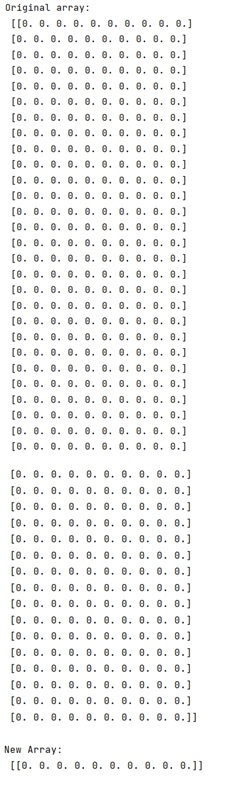# NumPy index slice without losing dimension information

Learn, how to slice the index of NumPy array without losing the dimension information?
Submitted by Pranit Sharma, on January 05, 2023

NumPy is an abbreviated form of Numerical Python. It is used for different types of scientific operations in python. Numpy is a vast library in python which is used for almost every kind of scientific or mathematical operation. It is itself an array which is a collection of various methods and functions for processing the arrays.

## Slicing the index of NumPy array without losing the dimension information

The dimensionality of an array is preserved when indexing is performed by a list (or an array) of indexes. This is a good method because it allows us to make a choice between keeping the dimension and squeezing.

We will first slice all the fields and then we will double slice this array where we will use : to retain the dimensionality.

Let us understand with the help of an example,

## Python code to slice the index of NumPy array without losing the dimension information

```# Import numpy
import numpy as np

# Creating a numpy array
import numpy as np
arr = np.zeros((50,10))

# Display original array
print("Original array:\n",arr,"\n")

# Slicing array
res = arr[,:]

# Display result
print("New Array:\n",res)
```

Output: